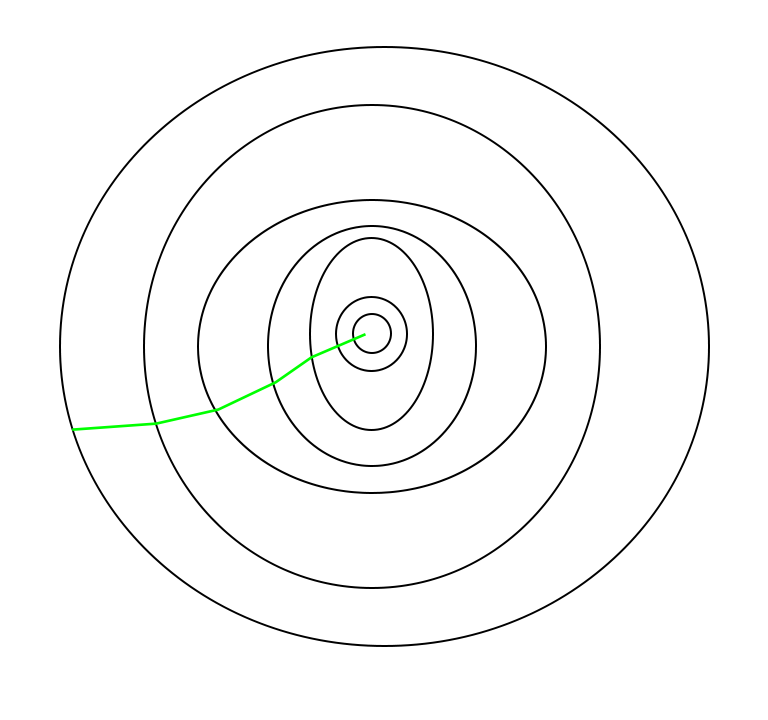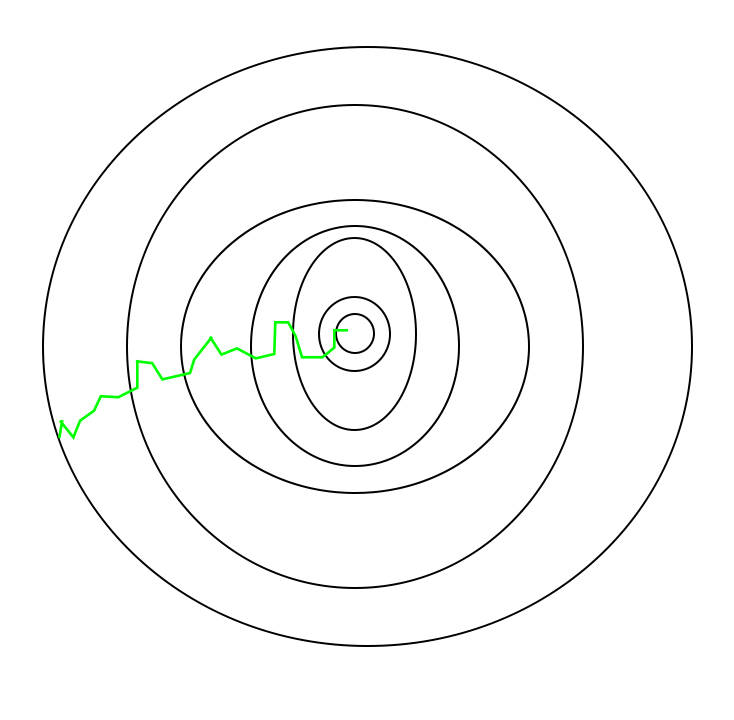GFG App
Open AppBrowser
Continue

# ML | Stochastic Gradient Descent (SGD)

Gradient Descent is an iterative optimization process that searches for an objective function’s optimum value (Minimum/Maximum). It is one of the most used methods for changing a model’s parameters in order to reduce a cost function in machine learning projects.

The primary goal of gradient descent is to identify the model parameters that provide the maximum accuracy on both training and test datasets. In gradient descent, the gradient is a vector pointing in the general direction of the function’s steepest rise at a particular point. The algorithm might gradually drop towards lower values of the function by moving in the opposite direction of the gradient, until reaching the minimum of the function.

Typically, there are three types of Gradient Descent:

Stochastic Gradient Descent (SGD) is a variant of the Gradient Descent algorithm that is used for optimizing machine learning models. It addresses the computational inefficiency of traditional Gradient Descent methods when dealing with large datasets in machine learning projects.

In SGD, instead of using the entire dataset for each iteration, only a single random training example (or a small batch) is selected to calculate the gradient and update the model parameters. This random selection introduces randomness into the optimization process, hence the term “stochastic” in stochastic Gradient Descent

The advantage of using SGD is its computational efficiency, especially when dealing with large datasets. By using a single example or a small batch, the computational cost per iteration is significantly reduced compared to traditional Gradient Descent methods that require processing the entire dataset.

• Initialization: Randomly initialize the parameters of the model.
• Set Parameters: Determine the number of iterations and the learning rate (alpha) for updating the parameters.
• Stochastic Gradient Descent Loop: Repeat the following steps until the model converges or reaches the maximum number of iterations:

a. Shuffle the training dataset to introduce randomness.

b. Iterate over each training example (or a small batch) in the shuffled order.

c. Compute the gradient of the cost function with respect to the model parameters using the current training                         example (or batch).

d. Update the model parameters by taking a step in the direction of the negative gradient, scaled by the                                 learning rate.

e. Evaluate the convergence criteria, such as the difference in the cost function between iterations of the                              gradient.

• Return Optimized Parameters: Once the convergence criteria are met or the maximum number of iterations is reached, return the optimized model parameters.

In SGD, since only one sample from the dataset is chosen at random for each iteration, the path taken by the algorithm to reach the minima is usually noisier than your typical Gradient Descent algorithm. But that doesn’t matter all that much because the path taken by the algorithm does not matter, as long as we reach the minimum and with a significantly shorter training time.

#### The path taken by Batch Gradient Descent is shown below:#### A path taken by Stochastic Gradient Descent looks as follows –One thing to be noted is that, as SGD is generally noisier than typical Gradient Descent, it usually took a higher number of iterations to reach the minima, because of the randomness in its descent. Even though it requires a higher number of iterations to reach the minima than typical Gradient Descent, it is still computationally much less expensive than typical Gradient Descent. Hence, in most scenarios, SGD is preferred over Batch Gradient Descent for optimizing a learning algorithm.

## Python Code For Stochastic Gradient Descent

We will create an SGD class with methods that we will use while updating the parameters, fitting the training data set, and predicting the new test data. The methods we will be using are as :

Gradient – This method will be used in updating the parameters of the model. For every iteration, it will calculate the error between the predicted data point and the actual data point.

Fit This method will be used to fit the training dataset into the machine learning model. It will shuffle the data indices and will calculate the gradient for each data point and update the parameter theta.

Predict – This method will be used to predict new data points. As the prediction is just the dot product of parameter and dataset elements.

## Python3

 `import` `numpy as np`   `class` `SGD:` `    ``def` `__init__(``self``, lr``=``0.01``, max_iter``=``1000``, batch_size``=``32``, tol``=``1e``-``3``):` `        ``# learning rate of the SGD Optimizer` `        ``self``.learning_rate ``=` `lr ` `        ``# maximum number of iterations for SGD Optimizer` `        ``self``.max_iteration ``=` `max_iter ` `        ``# mini-batch size of the data ` `        ``self``.batch_size ``=` `batch_size  ` `        ``# tolerance for convergence for the theta ` `        ``self``.tolerence_convergence  ``=` `tol  ` `        ``# Initialize model parameters to None` `        ``self``.theta ``=` `None`  `        `  `    ``def` `fit(``self``, X, y):` `        ``# store dimension of input vector ` `        ``n, d ``=` `X.shape` `        ``# Intialize random Theta for every feature ` `        ``self``.theta ``=` `np.random.randn(d)` `        ``for` `i ``in` `range``(``self``.max_iteration):` `            ``# Shuffle the data` `            ``indices ``=` `np.random.permutation(n)` `            ``X ``=` `X[indices]` `            ``y ``=` `y[indices]` `            ``# Iterate over mini-batches` `            ``for` `i ``in` `range``(``0``, n, ``self``.batch_size):` `                ``X_batch ``=` `X[i:i``+``self``.batch_size]` `                ``y_batch ``=` `y[i:i``+``self``.batch_size]` `                ``grad ``=` `self``.gradient(X_batch, y_batch)` `                ``self``.theta ``-``=` `self``.learning_rate ``*` `grad` `            ``# Check for convergence` `            ``if` `np.linalg.norm(grad) < ``self``.tolerence_convergence:` `                ``break` `    ``# define a gradient functon for calculating gradient ` `    ``# of the data ` `    ``def` `gradient(``self``, X, y):` `        ``n ``=` `len``(y) ` `        ``# predict target value by taking taking ` `        ``# taking dot product of dependent and theta value ` `        ``y_pred ``=` `np.dot(X, ``self``.theta)` `        `  `        ``# calculate error between predict and actual value ` `        ``error ``=` `y_pred ``-` `y` `        ``grad ``=` `np.dot(X.T, error) ``/` `n` `        ``return` `grad` `    `  `    ``def` `predict(``self``, X):` `        ``# prdict y value using calculated theta value ` `        ``y_pred ``=` `np.dot(X, ``self``.theta)` `        ``return` `y_pred`

### SGD Implementation

We will create a random dataset with 100 rows and 5 columns and we fit our Stochastic gradient descent Class on this data.  Also, We will use predict method from SGD

## Python3

 `# Create random dataset with 100 rows and 5 columns` `X ``=` `np.random.randn(``100``, ``5``)` `# create corresponding target value by adding random` `# noise in the dataset` `y ``=` `np.dot(X, np.array([``1``, ``2``, ``3``, ``4``, ``5``]))\` `    ``+` `np.random.randn(``100``) ``*` `0.1` `# Create an instance of the SGD class` `model ``=` `SGD(lr``=``0.01``, max_iter``=``1000``,` `            ``batch_size``=``32``, tol``=``1e``-``3``)` `model.fit(X, y)` `# Predict using predict method from model` `y_pred ``=` `model.predict(X)`

This cycle of taking the values and adjusting them based on different parameters in order to reduce the loss function is called back-propagation.

Speed: SGD is faster than other variants of Gradient Descent such as Batch Gradient Descent and Mini-Batch Gradient Descent since it uses only one example to update the parameters.

Memory Efficiency: Since SGD updates the parameters for each training example one at a time, it is memory-efficient and can handle large datasets that cannot fit into memory.

Avoidance of Local Minima: Due to the noisy updates in SGD, it has the ability to escape from local minima and converges to a global minimum.